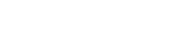# math.roundhalfdown

`FLOATmath.roundhalfdownFLOATx`

Available inall subroutines.

Rounds to nearest, ties towards negative infinity (also known as half down).

## Return Value

If `x` is `math.NAN`, a NaN will be returned.

If `x` is integral, ±0, `x` itself is returned.

If `x` is `math.POS_INFINITY` or `math.NEG_INFINITY`, an infinity of the same sign is returned.

Otherwise, the rounded value of `x` is returned.

BETA